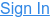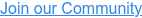Home Blog Find the latest reported values with ease. Introducing max_by and min_by aggregations in CrateDB v5.2

# CrateDB v5.2 adds two new aggregation functions: max_by and min_by

These aggregation functions allow users to quickly and easily search the value of one column based on the minimum or maximum value of another column, making them useful for analyzing trends, identifying outliers, or simply understanding the range of values within a dataset. An example use case is getting the latest measurement by using the time column and max_by(measurement, time).

`MIN_BY` and `MAX_BY` functions allow you to find the minimum or maximum value in a given column based on the values in another column. For example, if you have a table with two columns, product, category and price, you can use the min_by(product, price) to find the row with the product with the lowest price in each category:

``SELECT min_by(product, price) AS cheapest_product``FROM product_list``GROUP BY category;``

`max_by(returned_value, maximized_value)` and `min_by(returned_value, minimized_value)` return the value of the first column for which the value of the second column is maximized or minimized. If multiple rows maximize or minimize the result of the second column, the output will be non-deterministic and CrateDB can return any value from the list of resulting rows.

Both `max_by` and `min_by `can be used for numerical and non-numerical data.

Let’s start with examples using the dataset about power consumption. First, create a table with the schema below:

`CREATE TABLE IF NOT EXISTS doc.power_consumption (`
`   "ts" TIMESTAMP WITH TIME ZONE,`
`   "Global_active_power" REAL,`
`   "Global_reactive_power" REAL,`
`   "Voltage" REAL,`
`   "Global_intensity" REAL,`
`   "Sub_metering_1" REAL,`
`   "Sub_metering_2" REAL,`
`   "Sub_metering_3" REAL,`
`   "meter_id" TEXT,`
`   "location" GEO_POINT,`
`   "city" TEXT`
`);`

To import data, use the following` COPY FROM` command:

`COPY doc.power_consumptionFROM 'https://srv.demo.crate.io/datasets/power_consumption.json'RETURN SUMMARY;`

The dataset illustrates the consumption data for a few years and shows the differences between several measured utilities. For instance, the column `"Sub_metering_1" `shows how much energy is consumed in the kitchen. Similarly, columns `"Sub_metering_2"` and `"Sub_metering_3"` show the consumed energy in laundry and climate control systems. The full description of the dataset can be found here.

### Example queries

Given the data set, let’s find the ids of house meters that had the highest consumption at one point in time for the kitchen and laundry:

`SELECT max_by(meter_id, "Sub_metering_1") as max_kitchen,  `
`       max_by(meter_id, "Sub_metering_2") as max_laundry  `
`FROM doc.power_consumption;`

The result of this query should contain the following meter ids:

`+-------------+-------------+`
`| max_kitchen | max_laundry |`
`+-------------+-------------+`
`| 84007B127R  | 840070504U  |`
`+-------------+-------------+`
`SELECT 1 row in set (7.423 sec)`

Another example would be to find the meter id for the house with the lowest unused power:

`SELECT min_by(meter_id, "Global_reactive_power") AS min_unused `
`FROM doc.power_consumption;`

The return value will tell us for which house meter we had the lowest value of unused power:

`+------------+`
`| min_unused |`
`+------------+`
`| 84007B008L |`
`+------------+`
`SELECT 1 row in set (0.197 sec)`

You can also combine these functions with `WHERE` or `GROUP BY` clauses in CrateDB. For example, let's find for each meter id, the consumption of other, unmapped appliances when the unused power was the lowest:

`SELECT`
`  meter_id,`
`  min_by("Global_active_power","Global_reactive_power") AS total_consumption `
`FROM doc.power_consumption `
`GROUP BY meter_id `
`LIMIT 10;`

The query result will list the consumption for each meter id:

`+------------+-------------------+`
`| meter_id   | total_consumption |`
`+------------+-------------------+`
`| 840073190N |             0.202 |`
`| 840071457E |             0.258 |`
`| 840072897V |             0.14  |`
`| 840072655G |             0.218 |`
`| 840072219H |             0.274 |`
`| 840071893D |             1.342 |`
`| 840075260N |             0.246 |`
`| 840076398A |             0.226 |`
`| 840072328B |             0.212 |`
`| 840071760J |             0.222 |`
`+------------+-------------------+`
`SELECT 10 rows in set (0.067 sec)`

### Performance and alternatives

As you have seen above `min_by` and `max_by` provide a very concise and convenient way to easily find the value of one column based on the minimum or maximum value of another column. Not only is it a convenient feature, but it also provides significant performance gains to alternative queries one had to write in earlier versions of CrateDB.

Let us look at another example and see how much easier and faster it is to get the right results in CrateDB 5.2. We start with our often-used dataset containing IoT device data:

`CREATE TABLE IF NOT EXISTS devices.readings ( `
`       "time" TIMESTAMP WITH TIME ZONE NOT NULL, `
`       device_id TEXT, `
`       battery_level BIGINT, `
`       battery_status TEXT`
`    ) CLUSTERED BY (device_id) INTO 8 SHARDS;`

We want to find the latest reported `battery_level` and `battery_status` for each device in our dataset holding 30 Million total records. In CrateDB 5.1 and earlier versions, one could fallback to a 2-step approach and use a `JOIN` like so:

`SELECT`
`    r.device_id,`
`    r.time,`
`    r.battery_level,`
`    r.battery_temperature`
`FROM devices.readings r`
`    JOIN (SELECT`
`            MAX(time) time,`
`            device_id`
`        FROM devices.readings`
`        GROUP BY device_id) max_r`
`    ON max_r.time = r.time`
`    AND max_r.device_id = r.device_id;`

Not only makes the nested structure query adjustments more difficult and one needs to remember the pattern, but also the performance - due to the expensive `JOIN` - is not really that great with a runtime of roughly 9 seconds:

`Runtime (in ms):`
`    mean:    8982.507 ± 57.494`
`    min/max: 8578.380 → 9843.830`

That is an improvement of 85% in query speed (8.9s → 1.3s), also using a simpler syntax.

### Wrap up

Overall, the `max_by `and `min_by` functions in CrateDB provide an easy and efficient way to find the maximum or minimum value of a given column in a table based on the values in a different column. These functions can be used in a variety of scenarios to quickly and easily find the highest or lowest values in a set of data.

If you like this blog post and want to learn more about CrateDB, check out our documentation and join the CrateDB community!